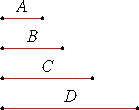# Definition 8

A proportion in three terms is the least possible.

# Definition 9

When three magnitudes are proportional, the first is said to have to the third the duplicate ratio of that which it has to the second.

# Definition 10

When four magnitudes are continuously proportional, the first is said to have to the fourth the triplicate ratio of that which it has to the second, and so on continually, whatever be the proportion.

## Guide

 In the illustration A, B, and C form three terms for the proportion A : B = B : C, therefore the ratio A : C is the duplicate ratio of A : B. For a numerical example, 4:9 is the duplicate ratio of 2:3. The illustration also shows a continued proportion of four magnitudes, A, B, C, and D, since A : B = B : C = C : D. Also, A : D is the triplicate ratio of A : B. For a numerical example, 8 : 27 is the triplicate ratio of 2 : 3.#### Continued proportions, also known as geometric progressions

In the second book on number theory, Book VIII, Euclid considers continued numeric proportions of arbitrary length such as

64, 96, 144, 316, 324, 486

See, for example, VIII.1. These are commonly called geometric progressions or geometric sequences. In a geometric progression, the ratio of each term to the next term is the same. Euclid finds the sum of numerical geometric progression in IX.35.